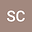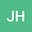loading page

New Blow-up Conditions to $p$-Laplace Type Nonlinear Parabolic Equations under Nonlinear Boundary Conditions
••• Soon-Yeong Chung,
• Jaeho Hwang
Soon-Yeong Chung
Sogang University College of Natural Sciences
Author ProfileJaeho Hwang
Sogang University College of Natural Sciences
Author Profile## Abstract

In this paper, we study blow-up phenomena of the following $$p$$-Laplace type nonlinear parabolic equations under nonlinear mixed boundary conditions and $$u=0$$ on $$\Gamma_{2}\times(0,t^{*})$$ such that $$\Gamma_{1}\cup\Gamma_{2}=\partial\Omega$$, where $$f$$ and $$h$$ are real-valued $$C^{1}$$-functions. To discuss blow-up solutions, we introduce new conditions:
For each $$x\in\Omega$$, $$z\in\partial\Omega$$, $$t>0$$, $$u>0$$, and $$v>0$$, for some constants $$\alpha$$, $$\beta_{1}$$, $$\beta_{2}$$, $$\gamma_{1}$$, $$\gamma_{2}$$, and $$\delta$$ satisfying where $$\rho_{m}:=\inf_{w>0}\rho(w)$$, $$P(v)=\int_{0}^{v}\rho(w)dw$$, $$F(x,t,u)=\int_{0}^{u}f(x,t,w)dw$$, and $$H(x,t,u)=\int_{0}^{u}h(x,t,w)dw$$. Here, $$\lambda_{R}$$ is the first Robin eigenvalue and $$\lambda_{S}$$ is the first Steklov eigenvalue for the $$p$$-Laplace operator, respectively.

#### Peer review status:Published

21 Aug 2020Submitted to Mathematical Methods in the Applied Sciences
21 Aug 2020Submission Checks Completed
21 Aug 2020Assigned to Editor
29 Aug 2020Reviewer(s) Assigned
07 Dec 2020Review(s) Completed, Editorial Evaluation Pending
08 Dec 2020Editorial Decision: Revise Minor
22 Dec 20201st Revision Received
22 Dec 2020Submission Checks Completed
22 Dec 2020Assigned to Editor
22 Dec 2020Editorial Decision: Accept
07 Feb 2021Published in Mathematical Methods in the Applied Sciences. 10.1002/mma.7172